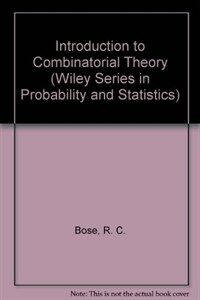> Detail View

# Detail View## Introduction to combinatorial theory

Material type
단행본
Personal Author
Bose, R. C. (Raj Chandra), 1901- Manvel, B. (Bennet), 1943-
Title Statement
Introduction to combinatorial theory / R.C. Bose and B. Manvel.
Publication, Distribution, etc
New York :   Wiley,   c1984.
Physical Medium
ix, 237 p. : ill. ; 24 cm.
Series Statement
Wiley series in probability and mathematical statistics.Probability and mathematical statistics, 0271-6232
ISBN
0471896144 :
Bibliography, Etc. Note
Includes bibliographical references and index.
Combinatorial analysis.
 000 00818camuuu200229 a 4500 001 000000072811 005 .0 008 830801s1984 nyua b 00110 eng 010 ▼a 83017019 //r84 020 ▼a 0471896144 : ▼c \$24.95 (est.) 049 ▼a l412616764 050 0 ▼a QA164 ▼b .B67 1984 082 0 ▼a 511/.6 ▼2 19 090 ▼a 512.5 ▼b B743i 100 1 0 ▼a Bose, R. C. ▼q (Raj Chandra), ▼d 1901- 245 1 0 ▼a Introduction to combinatorial theory / ▼c R.C. Bose and B. Manvel. 260 0 ▼a New York : ▼b Wiley, ▼c c1984. 300 ▼a ix, 237 p. : ▼b ill. ; ▼c 24 cm. 440 0 ▼a Wiley series in probability and mathematical statistics. ▼p Probability and mathematical statistics, ▼x 0271-6232 504 ▼a Includes bibliographical references and index. 650 0 ▼a Combinatorial analysis. 700 1 0 ▼a Manvel, B. ▼q (Bennet), ▼d 1943-

### Holdings Information

No. Location Call Number Accession No. Availability Due Date Make a Reservation Service
No. 1 Location Call Number 512.5 B743i Accession No. 412616764 Availability Available Due Date Make a Reservation Service

### Contents information

```CONTENTS
1. Permutations and Combinations = 1
1.1. Two Counting Principles = 1
1.2. Ordered Choices = 4
1.3. Unordered Choices = 7
1.4. Circular and Restricted Arrangements = 11
1.5. Finite Probability = 14
1.6. Compound Events and Expectations = 18
1.7. Remarks = 23
2. The Binomial Theorem = 24
2.1. The Binomial Theorem = 24
2.2. Binomial Identities = 27
2.3. Multinomial Coefficients and Negative Binomial Coefficients = 33
2.4. Binomial Probabilities = 38
2.5. Remarks = 42
3. Enumeration = 44
3.1. Fibonacci Numbers = 44
3.2. Linear Diophantine Equations = 47
3.3. Inclusion - Exclusion = 50
3.4. Partitions = 55
3.5. Generating Functions = 57
3.6. Recurrence Relations = 62
3.7. Remarks = 69
4. Graphs = 70
4.1. Introduction = 70
4.2. Basic Concepts = 73
4.3. Eulerian Trails = 77
4.4. Hamiltonian Graphs = 81
4.5. Trees = 87
4.6. Binary Trees = 91
4.7. Remarks = 98
5. Finite Fields = 99
5.1. Introduction = 99
5.2. The Galois Field, G FP = 102
5.3. The Commutative Ring G FP ［x］ = 105
5.4. The Galois Field G F Pn = 108
5.5. Primitive Elements of G F Pn = 111
5.6. Operation in G F Pn = 113
5.7. Remarks = 118
6. Finite Plane Geometries = 119
6.1. The Geometry EG(2, Pn ) = 119
6.2. Some Properties of the Geometry EG(2, Pn ) = 124
6.3. The Projective Geometry PG(2, Pn ) = 130
6.4. Remarks = 134
7. Orthogonal Latin Squares and Error Correcting Codes = 135
7.1. Latin Squares = 135
7.2. Complete Sets of Orthogonal Latin Squares = 139
7.3. Error - Correcting Codes = 144
7.4. Hamming One - Error Correcting Binary Codes = 149
7.5. Remarks = 153
8. Balanced Incomplete Block Designs = 155
8.1. Relations Between Parameters = 155
8.2. Incidence Matrix of a BIB Design = 158
8.3. Symmetric BIB Designs = 163
8.4. Orthogonal Series Designs = 167
8.5. Symmetrically Repeated Differences = 170
8.6. Steiner Triples = 174
8.7. Symmetric BIB Designs with r ＝ (v - 1)／2 = 177
8.8. The Residual and Derived of a Symmetric BIB Design = 179
8.9. Remarks = 182
9. Problems of Choice = 184
9.1. Introduction = 184
9.2. The Pigeonhole Principle = 185
9.3. Ramsey's Theorem = 187
9.4. Erd o ·· s and Szekeres' Theorem = 192
9.5. Parity = 195
9.6. Remarks = 200
10. Optimization = 202
10.1. Introduction = 202
10.2. The Marriage Theorem = 205
10.3. Applications = 211
10.4. Algorithms for Matchings = 216
10.5. Maximum Flows = 220
10.6. Remarks = 231
Index = 233```

김대수 (2022)

민만식 (2022)

장철원 (2021)